# Bull Call Spread Payoff, Break-Even and R/R

This page explains bull call spread profit and loss at expiration and the calculation of its maximum gain, maximum loss, break-even point and risk-reward ratio.

## Bull Call Spread Basic Characteristics

Bull call spread, also known as long call spread, is a bullish option strategy, typically done when a trader expects the underlying security to increase in price, but not too much. It has limited risk and limited upside potential.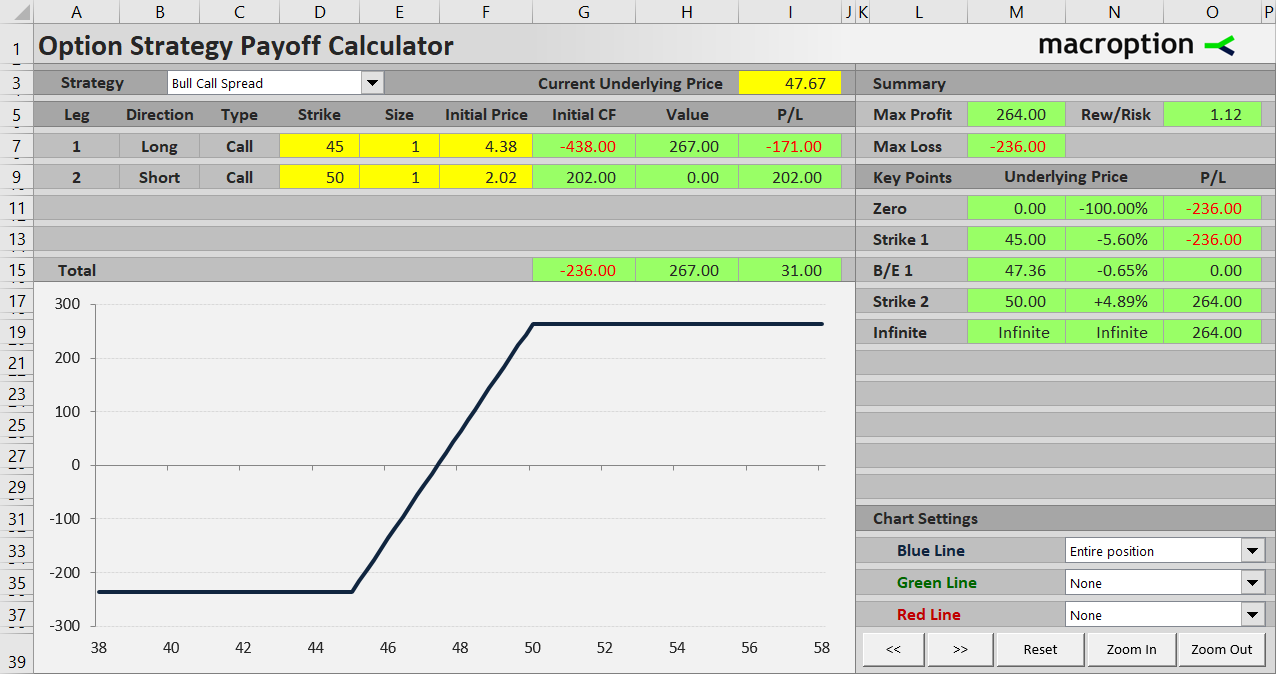A bull call spread position consists of two call options – buying a lower strike call and selling a higher strike call. It is a debit spread (negative cash flow when entering the position), because the price you pay for the lower strike call is typically higher than the price you get for selling the higher strike call.

We will explain the profit and loss profile and the calculation of maximum gain, risk and break-even point on an example.

Let's consider a bull call spread position created by the following transactions:

• Buy a \$45 strike call option for \$4.38 per share (after commissions), resulting in initial cash outflow of \$438 (assuming one option contract represents 100 shares of the underlying).
• Sell a \$50 strike call option with the same expiration date for net initial price of \$2.02, resulting in initial cash inflow of \$202.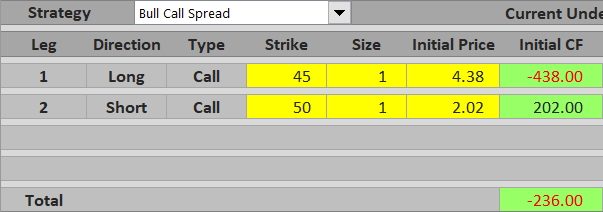The total initial cost is \$438 paid for the long call option minus \$202 received for the short call option, which is \$236.

The objective of a bull call spread trade is for the underlying price to increase before the options expire, so that our long call option ends up in the money by such amount that will offset the initial cost and make a profit. Assuming we hold the position until expiration, there are three possible scenarios.

## Scenario 1 (Maximum Loss)

The worst case scenario is that contrary to our expectations the underlying price declines and ends up below the lower strike price (in our example \$45). Both options expire worthless and there is zero cash flow at expiration. The total loss from the trade is equal to the initial cost, which is \$236 in our example.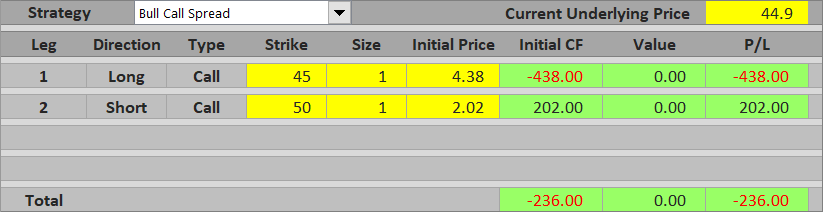It does not matter whether the underlying ends up just below \$45 or plummets to \$30. While our options are worthless at any price at or below \$45, we also can't lose more than what we have initially paid for the position.

Maximum possible loss, or risk of a bull call spread trade is equal to initial cost and applies when underlying price ends up below or exactly at the lower strike.

## Scenario 2 (Maximum Profit)

The ideal scenario is that the underlying price goes up and ends up at or above the higher strike at expiration. When this happens, both our call options are in the money.

Let's say the underlying ends up at \$52. We exercise the \$45 strike call and gain \$7 per share (\$52 minus \$45), or \$700. At the same time, we are assigned the \$50 call and lose \$2 per share (\$52 minus \$50), or \$200. Combining the two, we gain \$500 at expiration, or \$5 per share, which is exactly the difference between the two strikes. Because we initially paid \$236 for the position, our net profit from the trade is \$500 – \$236 = \$264.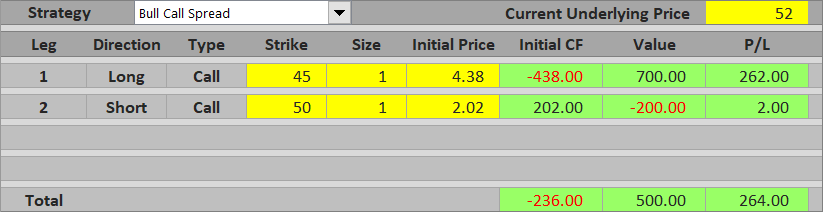Maximum possible profit from a bull call spread equals the difference between strikes (times number of shares) minus initial cost. It applies when the underlying ends up above or exactly at the higher strike.

## Scenario 3 (Between the Strikes)

So we know what happens when the underlying ends up below the lower strike (maximum loss) and above the higher strike (maximum profit). What if it ends up between the two strikes?

Below the higher strike the short call is out of the money. The outcome at expiration therefore depends entirely on the long lower strike call. For example, if underlying price is at \$47.67 at expiration, we will exercise the \$45 strike call and gain \$2.67 per share, or \$267. The \$50 strike call has no effect. Combined with the initial cost of \$236, the total profit from the trade is \$267 – \$236 = \$31.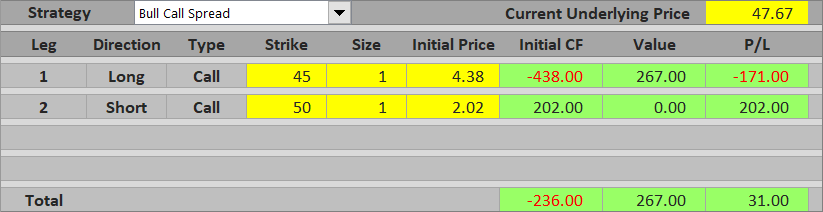The higher the underlying price gets above the lower strike, the greater the gain at expiration. As a result, the trade's profit or loss between the two strikes is increasing proportionally to underlying price. Near the lower strike it approaches maximum loss; near the higher strike it approaches maximum profit.

## Bull Call Spread Payoff Diagram

In the graph below you can see how the profit or loss behaves under the different scenarios and how the two options are driving it. The thick blue line represents overall P/L; the green line is the long \$45 strike call; the red line is the short \$50 call.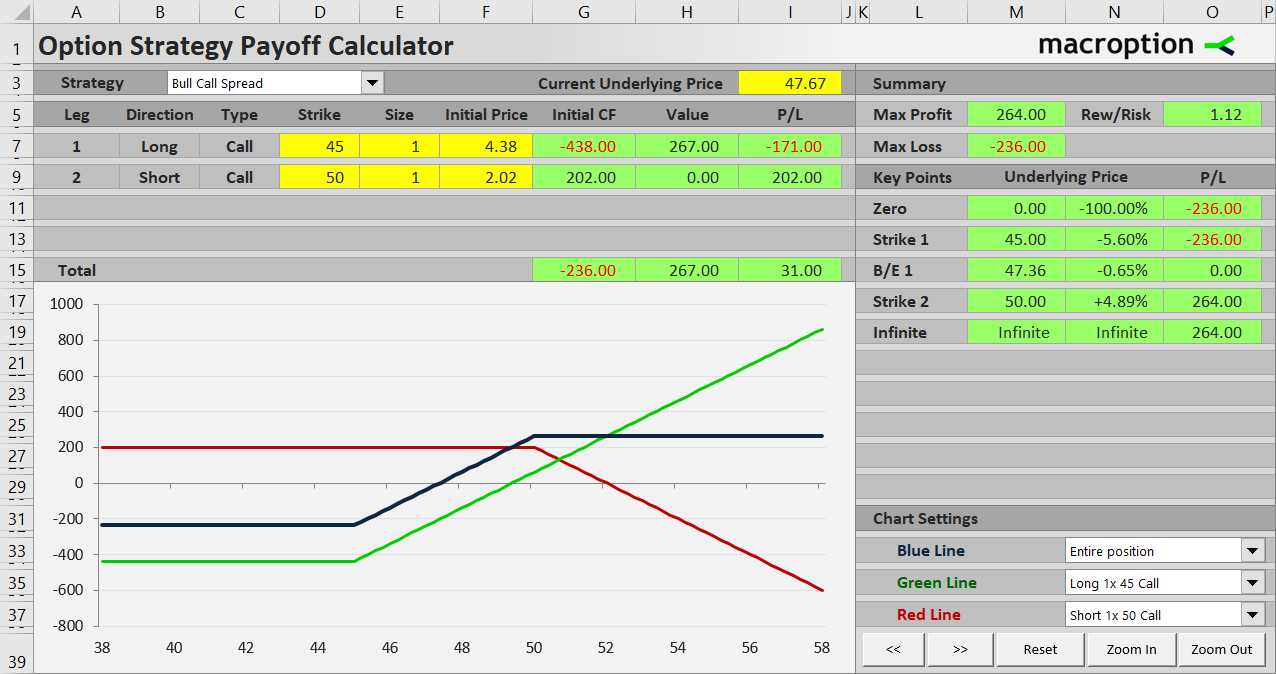Below \$45 the payoff is constant – a loss equal to initial cost of the position.

Between \$45 and \$50 it rises proportionally to underlying price due to increase in the long call option's value. The short call option is still out of the money.

Above \$50 the total P/L is constant and positive, as the lower strike call continues to increase in value, but this increase is now offset by the rising value of the short upper strike call.

## Bull Call Spread Break-Even Point

Besides the two strikes, the most important point in the chart is the moment when total payoff crosses zero and the trade starts being profitable – the break-even point. It is the underlying price at which the lower strike call option value is exactly equal to the initial cost of the entire position.

In our example the initial cost is \$236, or \$2.36 per share, and therefore the break-even point is at underlying price equal to \$45 + \$2.36 = \$47.36.

The general formula for bull call spread break-even point is:

B/E = lower strike + initial cost

## Bull Call Spread Risk-Reward Ratio

Knowing the maximum loss (scenario 1) and maximum profit (scenario 2) we can also calculate the risk-reward ratio. In our example, maximum loss is \$2.36 per share and maximum profit is \$2.64 per share. Risk-reward ratio is therefore 1:1.12.

Below you can see the general formulas:

Maximum loss (risk) = initial cost

Maximum profit (reward) = higher strike – lower strike – initial cost

Note that also:

Maximum profit (reward) = higher strike – B/E

## Similar Option Strategies

Bull call spread profit and loss profile is very similar to bull put spread. The difference is obviously that the latter uses puts rather than calls and it is a credit spread (the position is entered with net positive initial cash flow).

Another strategy with similar, bullish payoff is collar. It is best used when you are already long the underlying stock and want to create an exposure similar to bull call spread (limited risk and limited upside).

Bull call spread and bull put spread payoff profiles are inverse to bear put spread and bear call spread, which as their names suggest are bearish strategies (profit when underlying price goes down). The latter is actually the exact other side of bull call spread (you sell the lower strike call and buy the higher strike call).

Bull call spread is also closely related to plain and simple long call, as both are bullish and have limited risk. Compared to long call with the same strike price as the spread's long call (lower) strike, bull call spread has lower initial cost (due to the cash you receive for selling the higher strike call) and therefore lower break-even point (therefore higher probability of profit, other things being equal). For these benefits you of course pay with limited upside. Therefore, if you think the underlying price might jump substantially, a long call might be a more suitable trade; if you think a greater increase in price is unlikely, a bull call spread might offer lower cost and better odds.

By remaining on this website or using its content, you confirm that you have read and agree with the Terms of Use Agreement.

We are not liable for any damages resulting from using this website. Any information may be inaccurate or incomplete. See full Limitation of Liability.

Content may include affiliate links, which means we may earn commission if you buy on the linked website. See full Affiliate and Referral Disclosure.

We use cookies and similar technology to improve user experience and analyze traffic. See full Cookie Policy.